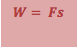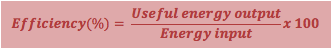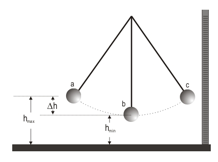# Work, Energy and Power

What is energy?

·      It is very difficult to define

·      Energy difference is a quantity that it is possible to calculate for any physical interaction

·      It is an indication as to whether that interaction is possible or not

·      It is helpful to think of some objects as energy stores e.g. a battery, a flywheel

·      It is synonymous with the term work, which is when a force makes an object move

·      It is measured in units called Joules (J) which is defined as the energy transferred when a 1N force moves an object through a distance of 1m

Conservation of EnergyWork

·      Work is done (or energy transferred) when forces act e.g.

-     when a ball is lifted up, work is done against gravity

-     when a book is pushed along a desk, work is done against gravityPower

·      Power is the rate at which energy can be transferred e.g. a light bulb transfers electrical energy into heat and light. A 60W bulb transfers 60J per second, a 100W bulb is able to do it at a higher rate of 100J per second therefore we observe more light per second and the light is brighter

·      The units of power are Joules per second (Js-1) or Watts (W)

·      It is common to use kW or MW (1kW = 1 x 103 W, 1MW = 1 x 106 W)

·      Normally the equation for power is Energy divided by time but this can be usefully expressed in the equation below when considering how forces transfer energy. Can you show that this formula is correct?Efficiency·      No energy is ever lost in a transfer, but not all energy is converted into the forms we find useful. Some energy is wasted. The ratio of useful energy output to energy input is called the efficiency:

Kinetic Energy·      This is energy due to movement and it is given by:

Potential Energy

·      This is energy due to height (under gravity) and it is given by:Energy Changes Example